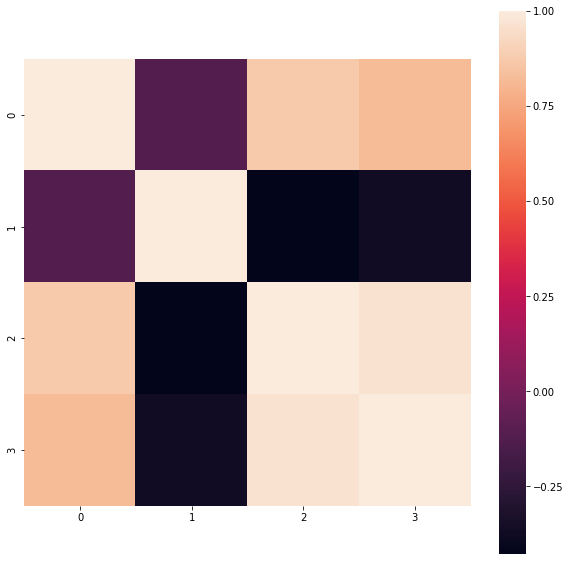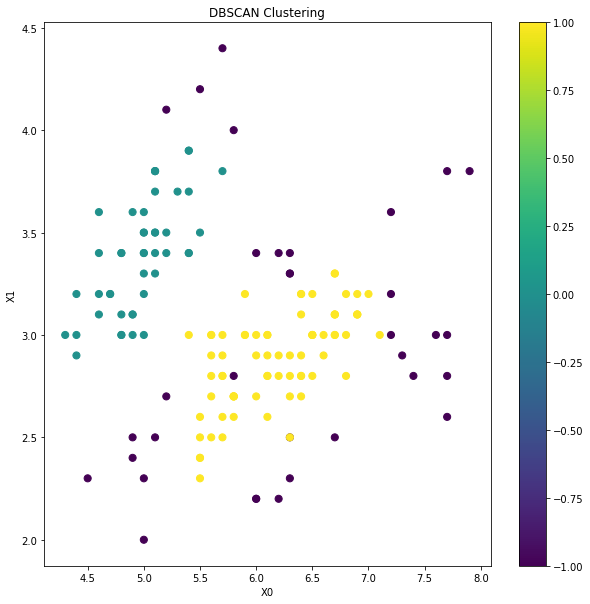How to do DBSCAN based Clustering in Python?
MACHINE LEARNING RECIPES DATA CLEANING PYTHON DATA MUNGING PANDAS CHEATSHEET     ALL TAGS

# How to do DBSCAN based Clustering in Python?

This recipe helps you do DBSCAN based Clustering in Python

0
In :
```## How to do DBSCAN based Clustering in Python
def Snippet_159():
print()
print(format('How to do DBSCAN based Clustering in Python','*^82'))

import warnings
warnings.filterwarnings("ignore")

from sklearn import datasets
from sklearn.preprocessing import StandardScaler
from sklearn.cluster import DBSCAN
import pandas as pd
import seaborn as sns
import matplotlib.pyplot as plt

X = iris.data; data = pd.DataFrame(X)
cor = data.corr()

fig = plt.figure(figsize=(10,10))
sns.heatmap(cor, square = True); plt.show()

# Standarize features
scaler = StandardScaler()
X_std = scaler.fit_transform(X)

# Conduct DBSCAN Clustering
clt = DBSCAN()

# Train model
model = clt.fit(X_std)

# Predict clusters
clusters = pd.DataFrame(model.fit_predict(X_std))
data['Cluster'] = clusters

# Visualise cluster membership
fig = plt.figure(figsize=(10,10)); ax = fig.add_subplot(111)
scatter = ax.scatter(data,data, c=data['Cluster'],s=50)
ax.set_title('DBSCAN Clustering')
ax.set_xlabel('X0'); ax.set_ylabel('X1')
plt.colorbar(scatter); plt.show()

Snippet_159()
```
```*******************How to do DBSCAN based Clustering in Python********************
```#### Relevant Projects

##### Predict Employee Computer Access Needs in Python
Data Science Project in Python- Given his or her job role, predict employee access needs using amazon employee database.

##### German Credit Dataset Analysis to Classify Loan Applications
In this data science project, you will work with German credit dataset using classification techniques like Decision Tree, Neural Networks etc to classify loan applications using R.

##### Ecommerce product reviews - Pairwise ranking and sentiment analysis
This project analyzes a dataset containing ecommerce product reviews. The goal is to use machine learning models to perform sentiment analysis on product reviews and rank them based on relevance. Reviews play a key role in product recommendation systems.

##### Sequence Classification with LSTM RNN in Python with Keras
In this project, we are going to work on Sequence to Sequence Prediction using IMDB Movie Review Dataset​ using Keras in Python.

##### Perform Time series modelling using Facebook Prophet
In this project, we are going to talk about Time Series Forecasting to predict the electricity requirement for a particular house using Prophet.

##### Machine Learning project for Retail Price Optimization
In this machine learning pricing project, we implement a retail price optimization algorithm using regression trees. This is one of the first steps to building a dynamic pricing model.

##### Data Science Project-TalkingData AdTracking Fraud Detection
Machine Learning Project in R-Detect fraudulent click traffic for mobile app ads using R data science programming language.

##### Solving Multiple Classification use cases Using H2O
In this project, we are going to talk about H2O and functionality in terms of building Machine Learning models.

##### PySpark Tutorial - Learn to use Apache Spark with Python
PySpark Project-Get a handle on using Python with Spark through this hands-on data processing spark python tutorial.

##### Customer Churn Prediction Analysis using Ensemble Techniques
In this machine learning churn project, we implement a churn prediction model in python using ensemble techniques.